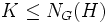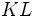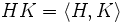# Subnormality is normalizing join-closed

This article gives the statement, and possibly proof, of a subgroup property (i.e., subnormal subgroup) satisfying a subgroup metaproperty (i.e., normalizing join-closed subgroup property)
View all subgroup metaproperty satisfactions | View all subgroup metaproperty dissatisfactions |Get help on looking up metaproperty (dis)satisfactions for subgroup properties
Get more facts about subnormal subgroup |Get facts that use property satisfaction of subnormal subgroup | Get facts that use property satisfaction of subnormal subgroup|Get more facts about normalizing join-closed subgroup property

## Statement

Suppose$H,K \le G$ are subnormal subgroups, with the property that$K \le N_G(H)$: in other words,$K$ normalizes$H$. Then the join of subgroups$\langle H, K \rangle$ is also subnormal. Moreover, the subnormal depth of$\langle H, K \rangle$ is bounded from above by the products of subnormal depths of$H$ and$K$.

## Facts used

1. Join of normal and subnormal implies subnormal of same depth: If$L$ is normal in$G$ and$K$ is$k$-subnormal in$G$, then$KL$ is subnormal in$G$ with subnormal depth at most$k$.
2. Normality is upper join-closed: If a subgroup is normal in two intermediate subgroups, it is normal in their join.
3. Subnormality satisfies intermediate subgroup condition: More specifically, if$A \le B \le G$ are groups such that$A$ is$k$-subnormal in$G$, then$A$ is also$k$-subnormal in$B$.
4. Subnormal subgroup has a unique fastest descending subnormal series, where the series members are obtained by taking successive normal closures.

## Proof

This proof uses a tabular format for presentation. Provide feedback on tabular proof formats in a survey (opens in new window/tab) | Learn more about tabular proof formats|View all pages on facts with proofs in tabular format

Given: A group$G$, subnormal subgroups$H, K \le G$ such that$K \le N_G(H)$, i.e.,$K$ normalizes$H$.$H$ has subnormal depth$h$ and$K$ has subnormal depth$k$.

To prove:$HK = \langle H, K \rangle$ is a subnormal subgroup, with subnormal depth at most$hk$.

Proof:

Step no. Assertion/construction Facts used Given data used Previous steps used Explanation
1 Consider the descending chain$G_i$ defined by$G_0 = G$, and$G_{i+1}$ is the normal closure of$H$ in$G_i$. This is the fastest descending subnormal series for$H$, and thus,$G_h = H$. Fact (4)$H$ is$h$-subnormal in$G$
2$K$ normalizes$G_i$ for all$i$. In particular, for any$i$,$\langle G_i, K \rangle = G_iK$.$K$ normalizes$H$ Step (1) Any subgroup of$G$ defined deterministically in terms of$H$ must be invariant under any automorphism that leaves$H$ invariant.
3 For each$i$,$G_{i+1}$ is normal in$G_iK$. Fact (2) Steps (1), (2) By construction,$G_{i+1}$ is normal in$G_i$, and as observed in Step (2),$K$ normalizes$G_{i+1}$, so$G_{i+1}$ is normal in$G_iK$ (fact (2)).
4 For each$i$,$K$ is$k$-subnormal in$G_iK$ Fact (3)$K$ is$k$-subnormal in$G$ Given-fact combination direct
5 For each$i$,$G_{i+1}K$ is$k$-subnormal in$G_iK$ Fact (1) Steps (3), (4) Step-fact combination direct
6$HK$ is$hk$-subnormal in$G$ Steps (1), (5) We have a chain:$HK = G_hK \le G_{h-1}K \le \dots \le G_1K \le G_0K = G$
where each member is$k$-subnormal in its successor. This tells us that$HK$ is$hk$-subnormal in$G$.